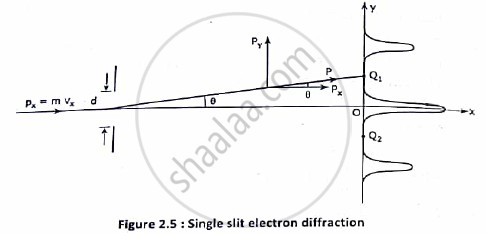Advertisement Remove all ads

# Arrive at Heisenberg’s uncertainty principle with single slit electron diffraction. An electron has a speed of 300n/sec with uncertainty of 0.01 %. Find the accuracy in its position. - Applied Physics 1

Answer in Brief

Arrive at Heisenberg’s uncertainty principle with single slit electron diffraction. An electron has a speed of 300n/sec with uncertainty of 0.01 %. Find the accuracy in its position.

Advertisement Remove all ads

#### Solution

SINGLE SLIT ELECTRON DIFFRACTION : THE WAVE CHARACTRISTICS OF AN ELECTRON
⦁ Consider an electron moving in ‘x’ direction with a velocity ‘Vx' and an initial momentum of px = mvx incident on a narrow slit of width ‘d’.
⦁ The electron is diffracted through an angle θ and strikes the screen the screen at point Q1 or point Q2 on either side of the central point O.

⦁ On the way to the screen the electron gains a y component of momentum ‘py'. As a result it  reaches the point Q1 with a resultant momentum of p.

⦁  it is seen that p = psinθ, which varies with the angle of diffraction θ.⦁ As there is no force acting in x-direction on the electron , p remains constant. Therefore the inaccuracy in the measurement of momentum arises from py
The maximum uncertainty in the measurement of momentum can be the momentum itself.
Therefore, ∆py = p = psinθ. …………………………………(1)

⦁ For θ small it can be assumed that Q
is the first minimum of the electron diffraction

pattern. In that case the condition for first minimum is:-
d sinθ = λ . …………………………………..(2)

⦁ From (1) and (2) it can be written as ∆p_y = pλ/ d ………………………………..(3)

⦁ On the other hand the electron needs to pass through any point of the slit , to be diffracted. Therefore the inaccuracy in determining the position of the electron in very small given by
∆y_m = d …………………………..(4)
⦁ From (3) and (4) it is found that

  ∆y_m ∆p_ yma = d.pλ/d = pλ

∆y_m.∆p _(yma) = h
This verifies the uncertainty principle.

NUMERICAL:-

Given Data  :- V = 300m/sec ,     ∆v /v= 0.01 %

Formula :- ∆x.∆p ≥ ħ
Calculations :- ∆x.m.∆p ≥ ħ

∆v = 300×(0.01)/(100) = 0.03

∆x ≥ ħ/ (m∆v) ≥ ( 6.63 ×10^( -34))/(2×3.14×9.1×0.03×10^(-31))

≥ 3.8 ×10-3
Therefore uncertainty in position = 3.8 ×10-3 m

Concept: Heisenberg’S Uncertainty Principle
Is there an error in this question or solution?
Advertisement Remove all ads

#### APPEARS IN

Advertisement Remove all ads
Advertisement Remove all ads
Share
Notifications

View all notifications

Forgot password?